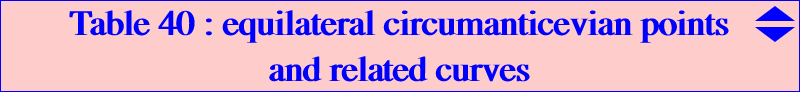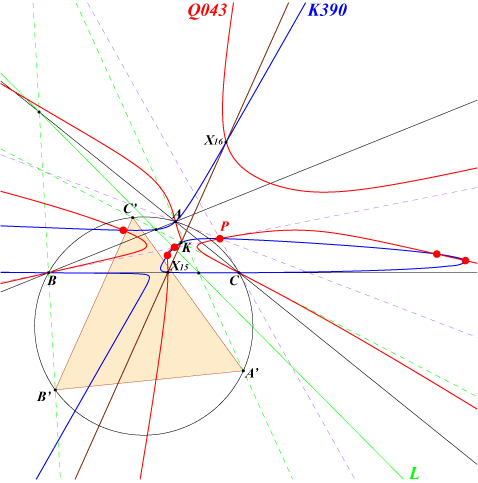Let P be a point and L its trilinear polar meeting the sidelines of ABC at U, V, W. The lines AU, BV, CW – these are the sidelines of the anticevian triangle of P – meet the circumcircle again at A', B', C' and the triangle A'B'C' is called circumanticevian triangle of P. See TCCT 7.19 p.201. When this triangle is equilateral, P is said to be an equilateral circumanticevian point. Theorem : the circumanticevian triangle of P and the anticevian triangle of the isogonal conjugate P* of P are similar. This shows that the equilateral circumanticevian points are the isogonal conjugates of the equilateral anticevian points we meet in Table 14. It easily follows that there are six (not neccessarily all real) equilateral circumanticevian points and that they lie on the isogonal transforms of all the curves seen in Table 14. See also the related Table 10. In particular, these points lie on K390 and Q043.